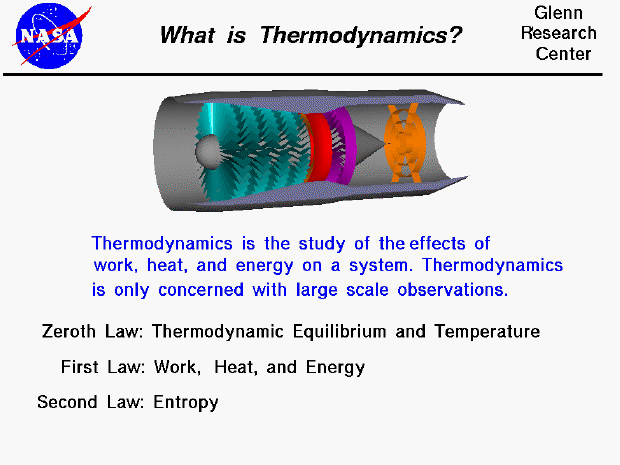Thermodynamics is a branch of physics which deals with the energy and work of a system. It was born in the 19th century as scientists were first discovering how to build and operate steam engines. As mentioned on the gas properties slide, thermodynamics deals only with the large scale response of a system which we can observe and measure in experiments. Small scale gas interactions are described by the kinetic theory of gasses which is a compliment to thermodynamics.

There are three principal laws of thermodynamics which are described on separate slides. Each law leads to the definition of thermodynamic properties which help us to understand and predict the operation of a physical system. We will present some simple examples of these laws and properties for a variety of physical systems, although, as aerodynamicists, we are most interested in thermodynamics in the study of propulsion systems and understanding high speed flows. Fortunately, many of the classical examples involve gas dynamics. Unfortunately, the numbering system for the three laws of thermodynamics is a bit confusing. We begin with the zeroth law.

The zeroth law of thermodynamics involves some simple definitions of thermodynamic equilibrium. Thermodynamic equilibrium leads to the large scale definition of temperature, as opposed to the small scale definition related to the kinetic energy of the molecules. The first law of thermodynamics relates the various forms of energy in a system (kinetic and potential) to the work which a system can perform and to the transfer of heat. This law is sometimes taken as the definition of internal energy, and introduces an additional state variable, enthalpy. The first law of thermodynamics allows for many possible states of a system to exist. But experience indicates that only certain states occurs. This leads to the second law of thermodynamics and the definition of another state variable called entropy. The second law stipulates that the total entropy of a system plus its environment can not decrease; it can remain constant for a reversible process but must always increase for an irreversible process.

Guided Tours